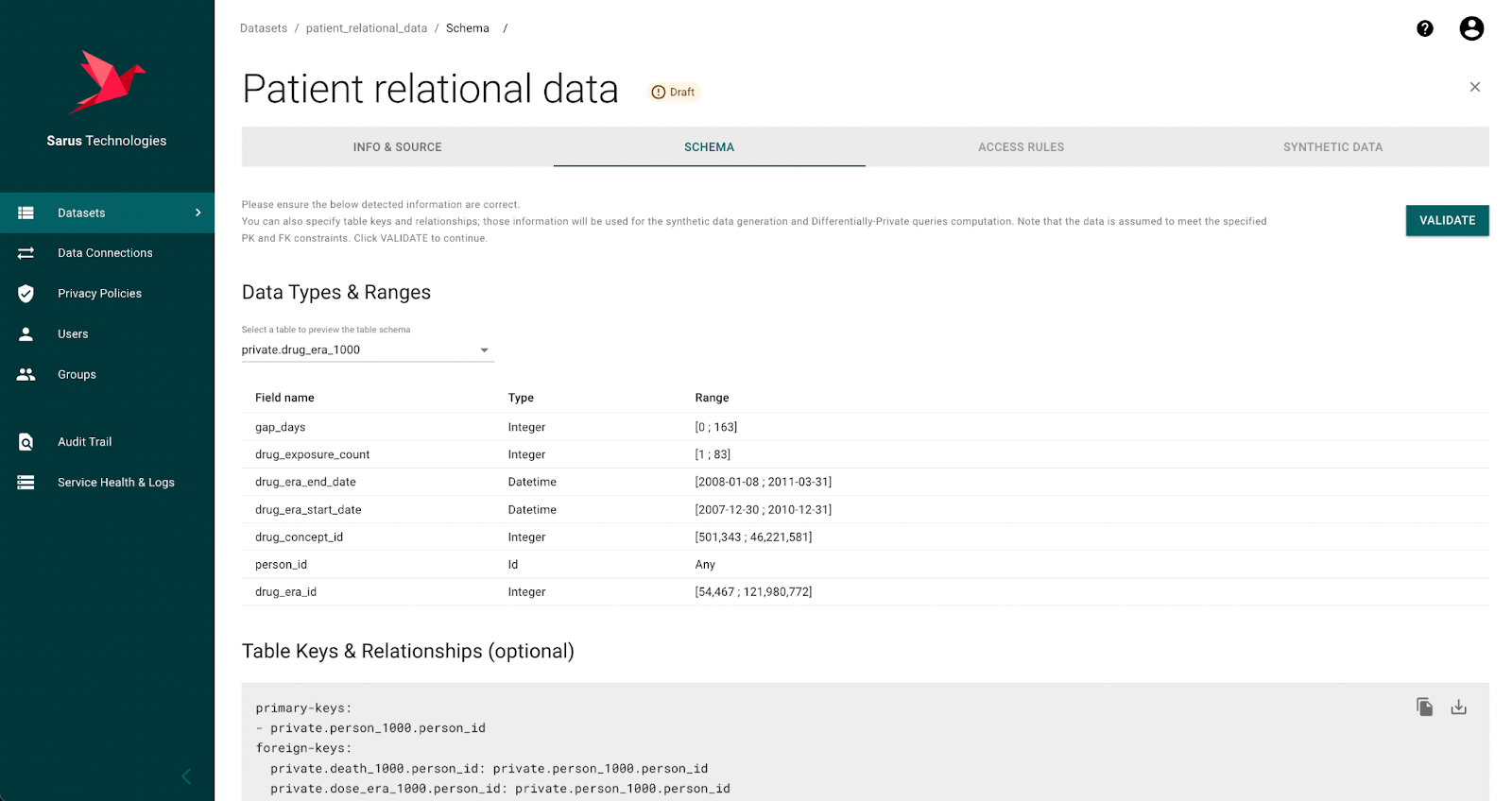PRODUCT UPDATE

# [Product update] Sarus supports relational tables!

The whole Sarus workflow now supports relational data for analysis with privacy guarantees.

Data Science
Marketing
Differential Privacy
Elodie Zanella## Introducing the support of relational tables

This new feature enables data consumers to leverage a data source made of several relational tables. Now, a data owner can grant access to an entire database without having to prepare a flat extract or apply any kind of data masking strategy. The data consumers are now able to leverage the full depth of the database, all that with privacy guarantees.

## Preserving privacy in relational data is a tough nut to crack

Intuitively, protecting privacy means that an individual’s information is kept secret. Differential privacy gives a more actionable definition of this but the intuition remains. In a table where one row is an individual, the objective is clear: the information of one row should not be leaked. But in relational databases, this is the exception and not the rule. To know how a row relates to an individual, one should parse the graph of foreign keys between tables to link each row of one table to a row of the user table. This is additional complexity already, but it is not the end of our story: the more foreign keys there are, the more likely the same individual corresponds to many rows in the table of interest. So now, we no longer need to make sure that one row is kept hidden, but also need to protect the block of all rows from one individual. And if things were not complex enough as it is, we also want to protect blocks of possible rows that are not in the data but could have been and, should they have been in the data, would have revealed user-level information.

So for Sarus, moving to multi-table support does not just mean that we are able to look up the name of the tables and adjust the SQL queries, it means that for every query or machine learning code that will run, we are able to assess the sensitivity of the output to adding or removing an individual to the database, including all the related rows in all the tables!

Luckily, differential privacy theoreticians have built the right framework to handle such complexity, it was time to implement it and make it user-friendly.

## What happens when onboarding a dataset with multiple tables with Sarus

When the data preparator onboards a dataset from a SQL database with multiple tables, the Sarus app retrieves the foreign key relationships. If the source does not support foreign keys (eg: BigQuery, Redshift, flat files), the data owner can define them manually.

During the onboarding process, Sarus will automatically generate a synthetic data version of the dataset. For multi-table datasets, it means both generating a fake table for each table and making sure the foreign keys are preserved in terms of relationships occurrences distribution. This way JOIN queries on synthetic data behave exactly like the same queries on the real data. As a result, analysts and data scientists get a real sense of the relational source.

Now the multi-table dataset is ready to be used. Let’s see it at work!

## ML on relational tables with Sarus Private Learning SDK

Let’s use a (public) patient dataset (OMOP) that has 7 tables. The data source is stored in a Postgre database and includes primary and foreign key constraints. We want to protect the privacy of each patient, their information is stored in the person table and all other tables point to the primary key — person_id — of this table.

Once onboarded with Sarus, we can manipulate this relational dataset with the Private Learning SDK (See this article for a full introduction to the SDK). You can find a Colab with the full analysis here.

Let’s extract the view we’re interested in:

```# Selecting remote patient dataset
remote_dataset = client.dataset(slugname="patient_relational_data")
print(remote_dataset.tables())
```
```# Extracting the SQL view of interest
extract = remote_dataset.sql("""SELECT *
FROM patient_relational_data.private.person_1000 AS patient
JOIN patient_relational_data.private.condition_occurrence_1000 AS cond_occ
ON patient.person_id = cond_occ.person_id
WHERE year_of_birth < 1942""")
df = extract.as_pandas()
print(df.shape)

We could also have built the extract in pandas. The synthetic rows of data, that preserves the relationships, helps a lot to do so!

Then we can preprocess the data and fit a ML model just as usual. Except the patient data is fully protected!

```# Handling types and NaNs
df = df.drop(['person_source_value','condition_source_value', 'condition_end_date', 'condition_start_date'], axis=1)
df = df.replace('', np.nan)
df = df.dropna(axis=1, how='all')
df.condition_source_concept_id = df.condition_source_concept_id.astype('int')

# Creating target
target = pd.DataFrame([1 if x==77670 else 0 for x in df['condition_concept_id']]).rename(columns={0: "target"})
df = pd.concat([df, target], axis=1)
df = df.drop(['condition_concept_id'], axis=1)
df = df.dropna()

```
```# Splitting into X,y
target_colname = 'target'
X = df.drop([target_colname], axis=1)
y = df[target_colname]

# Splitting into train and test datasets
result = train_test_split(X, y, test_size=0.3)
X_train = result
X_test = result
y_train = result
y_test = result

# Encoding y
lbl = LabelEncoder()
lbl.fit(y)

y_train = lbl.transform(y_train)
y_test = lbl.transform(y_test)
y = lbl.transform(y)

## Fitting model on remote preprocessed data
model = RandomForestClassifier()

fitted_model = model.fit(X=X_train, y=y_train)
y_pred = fitted_model.predict(X_test)

## Computing the accuracy
accuracy_score(y_pred, y_test)```

With this new relational data feature, data owners can grant access to entire databases and let the data consumers build the relevant extractions themselves. Data consumers get a real sense of the source data even without directly accessing it. This is a new step towards Sarus mission: let data lovers work on any data asset with privacy guarantees.

Want to try this feature in just a few minutes? Reach out!You're on the list! Thank you for signing up.
Oops! Something went wrong while submitting the form.32, rue Alexandre Dumas
75011 Paris — France
Blog